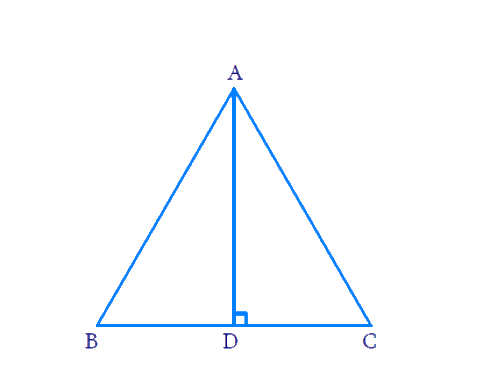# Ex.6.5 Q16 Triangles Solution - NCERT Maths Class 10

Go back to  'Ex.6.5'

## Question

In an equilateral triangle, prove that three times the square of one side is equal to four times the square of one of its altitudes.

DiagramWe have to prove $$3B{{C}^{2}}=4A{{D}^{2}}$$

## Text Solution

Reasoning :

In a right triangle, the square of the hypotenuse is equal to the sum of the squares of the other two sides.

Steps:

In $$\Delta ABC$$

$$AB = BC = CA$$

\begin{align}AD\bot BC\Rightarrow BD=CD=\frac{BC}{2}\end{align}

Now In $$\Delta ADC$$

\begin{align}&{A C^{2}=A D^{2}+C D^{2}} \\ &{B C^{2}=A D^{2}+\left(\frac{B C}{2}\right)^{2}\left[A C=B C \operatorname{and} C D=\frac{B C}{2}\right]} \\& {B C^{2}=A D^{2}+\frac{B C^{2}}{4}} \\& {B C^{2}-\frac{B C^{2}}{4}=A D^{2}} \\ &{\frac{3 B C^{2}}{4}=A D^{2}} \\ &{3 B C^{2}=4 A D^{2}}\end{align}

Learn from the best math teachers and top your exams

• Live one on one classroom and doubt clearing
• Practice worksheets in and after class for conceptual clarity
• Personalized curriculum to keep up with school